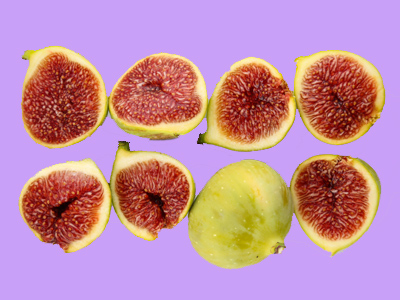8 can be divided into 2 groups of 4 or 4 groups of 2.

# Division and Sharing

This Math quiz is called 'Division and Sharing' and it has been written by teachers to help you if you are studying the subject at elementary school. Playing educational quizzes is an enjoyable way to learn if you are in the 3rd, 4th or 5th grade - aged 8 to 11.

It costs only \$12.50 per month to play this quiz and over 3,500 others that help you with your school work. You can subscribe on the page at Join Us

Division usually means sharing into equal groups. This can be related to repeated subtraction and pictures, representations, arrays or objects. These are often used to support this. Division facts can be related to the correct multiplication. For example: if a child knows that 2 x 4 = 8,
then they should recognize that 8 ÷ 4 = 2,
or 8 ÷ 2 = 4

This quiz will help children with divisions using representations and arrays.

Can you do division?

Question 1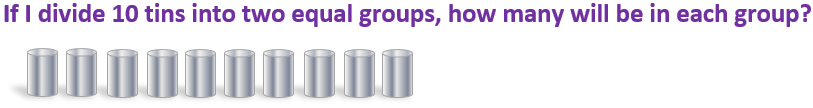8
11
10
5
10 ÷ 2 = 5
Question 2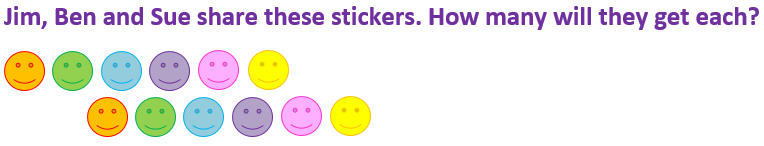12 x 2 = 24
12 ÷ 4 = 3
12 x 3 = 36
12 ÷ 3 = 4
There are 12 stickers to be shared between 3 people, so 12 ÷ 3 = 4
Question 3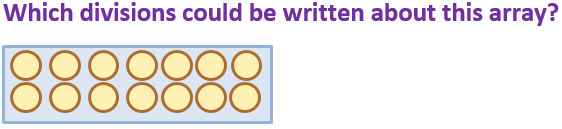16 ÷ 8 = 2 and 16 ÷ 2 = 8
10 ÷ 5 = 2 and 10 ÷ 2 = 5
12 ÷ 2 = 6 and 12 ÷ 6 = 2
14 ÷ 7 = 2 and 14 ÷ 2 = 7
There are 14 circles altogether
Question 4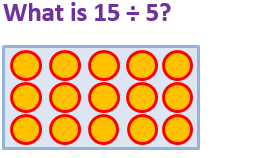35
5
15
3
15 shared into 5 equal groups gives 3 in each group
Question 5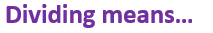Finding the total
Sharing into equal groups
Making numbers bigger
Dividing is the same as sharing into groups
Question 6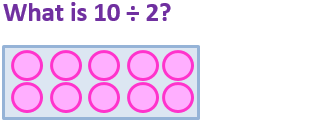10
5
2
20
10 ÷ 2 means sharing 10 into 2 equal groups, with 5 in each group
Question 7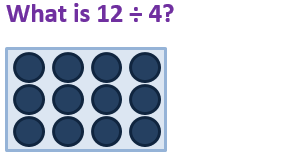3
4
12
8
12 ÷ 4 = 3 and 12 ÷ 3 = 4
Question 8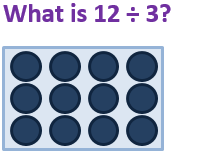30
12
8
4
12 shared into 3 equal groups gives 4 in each group
Question 9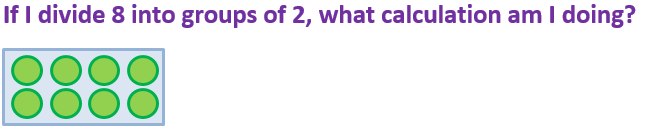8 ÷ 2
2 ÷ 8
16 ÷ 8
12 ÷ 2
Sharing into groups of 2 is the same as dividing by 2
Question 10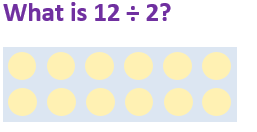22
16
6
12
12 shared into 2 equal groups is 6
Author:  Angela Smith